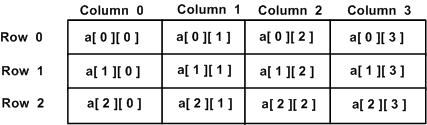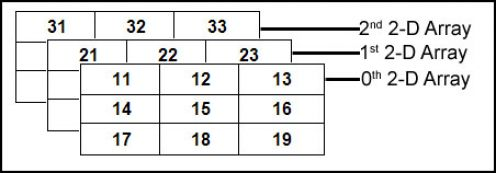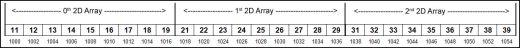# Multi-dimensional arrays

suggest change

The C programming language allows multidimensional arrays. Here is the general form of a multidimensional array declaration −

``type name[size1][size2]...[sizeN];``

For example, the following declaration creates a three dimensional (5 x 10 x 4) integer array:

``int arr;``

Two-dimensional Arrays

The simplest form of multidimensional array is the two-dimensional array. A two-dimensional array is, in essence, a list of one-dimensional arrays. To declare a two-dimensional integer array of dimensions m x n, we can write as follows:

``type arrayName[m][n];``

Where `type` can be any valid C data type (`int`, `float`, etc.) and `arrayName` can be any valid C identifier. A two-dimensional array can be visualized as a table with `m` rows and `n` columns. Note: The order does matter in C. The array `int a` is not the same as the array `int a`. The number of rows comes first as C is a row-major language.

A two-dimensional array `a`, which contains three rows and four columns can be shown as follows:Thus, every element in the array `a` is identified by an element name of the form `a[i][j]`, where `a` is the name of the array, `i` represents which row, and `j` represents which column. Recall that rows and columns are zero indexed. This is very similar to mathematical notation for subscripting 2-D matrices.

Initializing Two-Dimensional Arrays

Multidimensional arrays may be initialized by specifying bracketed values for each row. The following define an array with 3 rows where each row has 4 columns.

``````int a = {
{0, 1, 2, 3} ,   /*  initializers for row indexed by 0 */
{4, 5, 6, 7} ,   /*  initializers for row indexed by 1 */
{8, 9, 10, 11}   /*  initializers for row indexed by 2 */
};``````

The nested braces, which indicate the intended row, are optional. The following initialization is equivalent to the previous example:

``int a = {0,1,2,3,4,5,6,7,8,9,10,11};``

While the method of creating arrays with nested braces is optional, it is strongly encouraged as it is more readable and clearer.

Accessing Two-Dimensional Array Elements

An element in a two-dimensional array is accessed by using the subscripts, i.e., row index and column index of the array. For example −

``int val = a;``

The above statement will take the 4th element from the 3rd row of the array. Let us check the following program where we have used a nested loop to handle a two-dimensional array:

``````#include <stdio.h>

int main () {

/* an array with 5 rows and 2 columns*/
int a = { {0,0}, {1,2}, {2,4}, {3,6},{4,8}};
int i, j;

/* output each array element's value */
for ( i = 0; i < 5; i++ ) {

for ( j = 0; j < 2; j++ ) {
printf("a[%d][%d] = %d\n", i,j, a[i][j] );
}
}

return 0;
}``````

When the above code is compiled and executed, it produces the following result:

``````a: 0
a: 0
a: 1
a: 2
a: 2
a: 4
a: 3
a: 6
a: 4
a: 8``````

Three-Dimensional array:

A 3D array is essentially an array of arrays of arrays: it’s an array or collection of 2D arrays, and a 2D array is an array of 1D arrays.3D array memory map:Initializing a 3D Array:

``````double cprogram={
{{-0.1, 0.22, 0.3, 4.3}, {2.3, 4.7, -0.9, 2}},
{{0.9, 3.6, 4.5, 4}, {1.2, 2.4, 0.22, -1}},
{{8.2, 3.12, 34.2, 0.1}, {2.1, 3.2, 4.3, -2.0}}
};``````

We can have arrays with any number of dimensions, although it is likely that most of the arrays that are created will be of one or two dimensions.